# Civil Engineering - Waste Water Engineering

### Exercise :: Waste Water Engineering - Section 2

26.

For a circular sewer of diameter D running partially full with central angle α,

 A.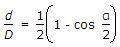B.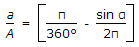C.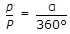D.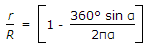E. all the above.

Explanation:

No answer description available for this question. Let us discuss.

27.

For design purposes, the normal rate of infiltration of ground water into the sewer, is

 A. 500 litres/km/cm B. 1000 litres/km/cm C. 1500 litres/km/cm D. 2000 litres/km/cm E. 2500 litres/km/cm.

Explanation:

No answer description available for this question. Let us discuss.

28.

The effluent of a septic tank is

 A. fit for discharge into any open drain B. foul and contains dissolved and suspended solids C. as good as that from a complete treatment D. none of these.

Explanation:

No answer description available for this question. Let us discuss.

29.

The drainage area of a town is 12 hectares. Its 40% area is hard pavement (K = 0.85), the 40% area is unpaved streets (K = 0.20) and the remaining is wooded areas (K = 0.15). Assuming the time of concentration for the areas as 30 minutes and using the formula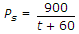the maximum run off is

 A. 0.10 cumec B. 0.12 cumec C. 0.15 cumec D. 0.20 cumec.

Explanation:

No answer description available for this question. Let us discuss.

30.

The detention period for plain sedimentation water tanks, is usually

 A. 4 to 8 hours B. 8 to 16 hours C. 16 to 24 hours D. 24 to 36 hours.